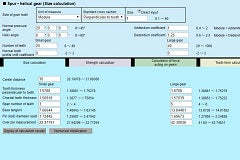# Gear calculator

The gear calculator is a comprehensive software which, after inputting various parameters related to gear calculations, computes on-line automatically gear sizes, strengths, working forces, tooth forms, backlash conversions, etc.

Because gear calculations require many complex formulas related to strengths and sizes, traditional gear design situations require highly specialized knowledge and suitable design time.

By utilizing the gear calculator introduced here, it is possible to reduce these burdens substantially. Among the parameters requiring user input are the unit to specify gear tooth such as module or diametral pitch (DP) and its value, pressure angle, helix angle, number of teeth, coefficient of profile shift, center distance, material, rpm (rotational speed), face width, safety ratio, circumferential backlash, etc.

This gear calculator software also handles gear calculations of various types of gears such as spur gears, worm gears, gear racks, bevel gears, internal gears, screw gears, etc.

Gear Calculator: https://khkgears.net/new/gear_calculator.html